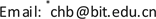1北京理工大学深圳研究院，广东 深圳

2北京理工大学光电学院光机电工程联合研究中心，北京1. 引言

2. 喷头体结构和磁场优化

B z 1 = B p + B c = J ∫ r R 0 R ( d N − z 1 ) d R [ R 2 + ( d N − z 1 ) 2 ] 3 / 2 + J t g 2 θ ∫ r / t g θ R 0 / t g θ θ z d z ( z − z 1 ) [ z 2 t g 2 θ + ( z − z 1 ) 2 ] 3 / 2 (1)

B 0 = J [ ( 1 − cos θ ) + sin 2 θ cos θ ln ( R 0 / r ) ] (2)

H M = 1 S m _ z o n e ⋅ N e I l m _ s l e e v e μ m _ s l e e v e S m _ s l e e v e + l m _ n o z z l e μ m _ n o z z l e S m _ n o z z l e + l m _ z o n e μ m _ z o n e S m _ z o n e (4)

Material and structure parameters of nozzl

3. 材料去除稳定性研究

4. 对复杂光滑曲面加工实验

5. 结语